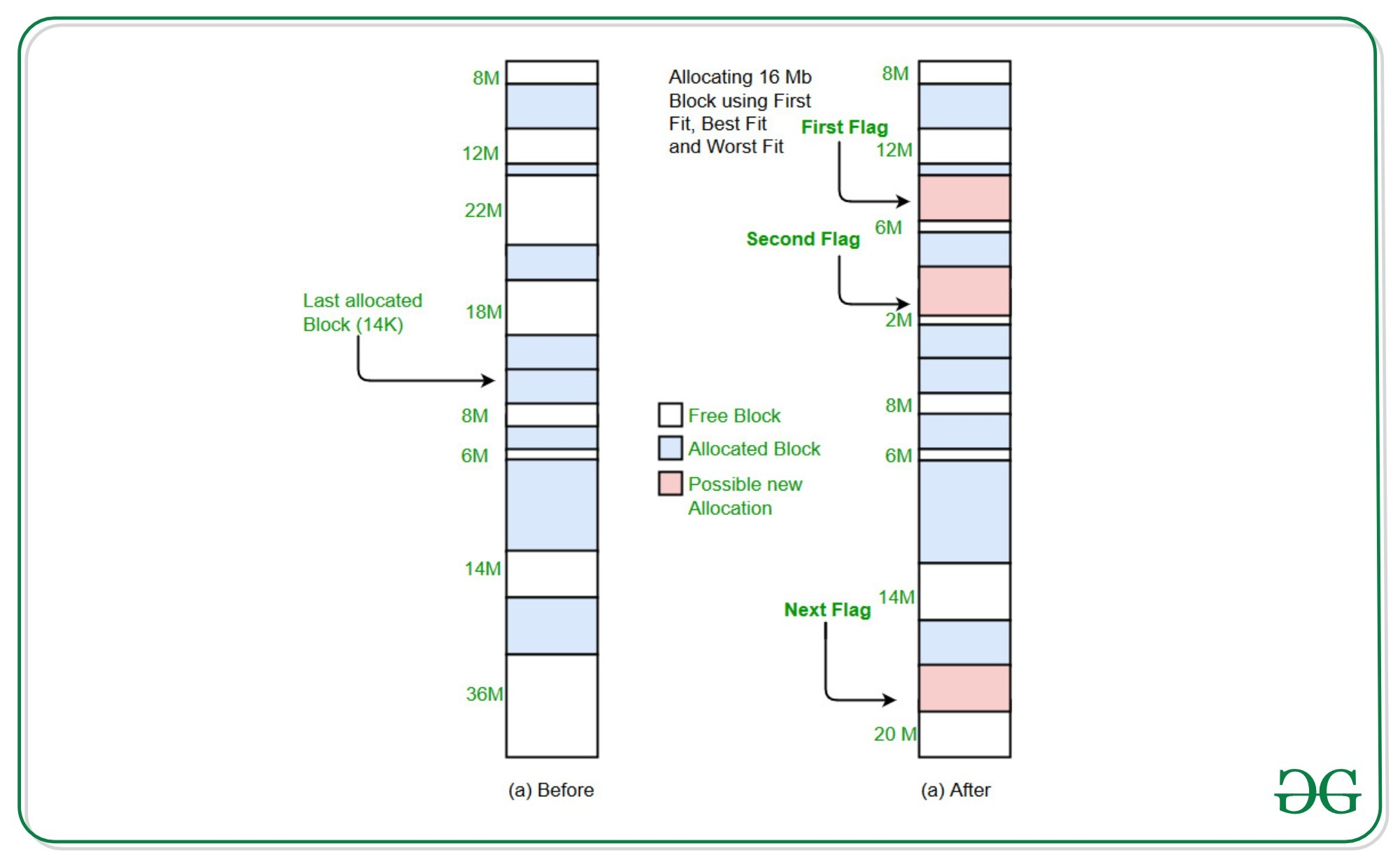Related Articles

# First Fit algorithm in Memory Management using Linked List

• Difficulty Level : Expert
• Last Updated : 17 Aug, 2020

First Fit Algorithm for Memory Management: The first memory partition which is sufficient to accommodate the process is allocated.

We have already discussed first fit algorithm using arrays in this article. However, here we are going to look into another approach using a linked list where the deletion of allocated nodes is also possible.

Attention reader! Don’t stop learning now. Get hold of all the important DSA concepts with the DSA Self Paced Course at a student-friendly price and become industry ready.  To complete your preparation from learning a language to DS Algo and many more,  please refer Complete Interview Preparation Course.

In case you wish to attend live classes with experts, please refer DSA Live Classes for Working Professionals and Competitive Programming Live for Students.

Examples:

```Input: blockSize[] = {100, 500, 200}
processSize[] = {417, 112, 426, 95}
Output:
Block of size 426 can't be allocated
Tag    Block ID    Size
0         1        417
1         2        112
2         0        95
After deleting block with tag id 0.
Tag    Block ID    Size
1         2        112
2         0        95
3         1        426
```## Recommended: Please try your approach on {IDE} first, before moving on to the solution.

Approach: The idea is to use the memory block with a unique tag id. Each process of different sizes are given block id, which signifies to which memory block they belong to, and unique tag id to delete particular process to free up space. Create a free list of given memory block sizes and allocated list of processes.

Create allocated list:
Create an allocated list of given process sizes by finding the first memory block with sufficient size to allocate memory from. If the memory block is not found, then simply print it. Otherwise, create a node and add it to the allocated linked list.

Delete process:
Each process is given a unique tag id. Delete the process node from the allocated linked list to free up some space for other processes. After deleting, use the block id of the deleted node to increase the memory block size in the free list.

Below is the implementation of the approach:

## C/C++

 `// C++ implementation of the First``// sit memory management algorithm``// using linked list`` ` `#include ``using` `namespace` `std;`` ` `// Two global counters``int` `g = 0, k = 0;`` ` `// Structure for free list``struct` `free` `{``    ``int` `tag;``    ``int` `size;``    ``struct` `free``* next;``}* free_head = NULL, *prev_free = NULL;`` ` `// Structure for allocated list``struct` `alloc {``    ``int` `block_id;``    ``int` `tag;``    ``int` `size;``    ``struct` `alloc* next;``}* alloc_head = NULL, *prev_alloc = NULL;`` ` `// Function to create free``// list with given sizes``void` `create_free(``int` `c)``{``    ``struct` `free``* p = (``struct` `free``*)``        ``malloc``(``sizeof``(``struct` `free``));``    ``p->size = c;``    ``p->tag = g;``    ``p->next = NULL;``    ``if` `(free_head == NULL)``        ``free_head = p;``    ``else``        ``prev_free->next = p;``    ``prev_free = p;``    ``g++;``}`` ` `// Function to print free list which``// prints free blocks of given sizes``void` `print_free()``{``    ``struct` `free``* p = free_head;``    ``cout << ``"Tag\tSize\n"``;``    ``while` `(p != NULL) {``        ``cout << p->tag << ``"\t"``             ``<< p->size << ``"\n"``;``        ``p = p->next;``    ``}``}`` ` `// Function to print allocated list which``// prints allocated blocks and their block ids``void` `print_alloc()``{``    ``struct` `alloc* p = alloc_head;``    ``cout << ``"Tag\tBlock ID\tSize\n"``;``    ``while` `(p != NULL) {``        ``cout << p->tag << ``"\t  "``             ``<< p->block_id << ``"\t\t"``             ``<< p->size << ``"\n"``;``        ``p = p->next;``    ``}``}`` ` `// Function to allocate memory to``// blocks as per First fit algorithm``void` `create_alloc(``int` `c)``{``    ``// create node for process of given size``    ``struct` `alloc* q = (``struct` `alloc*)``        ``malloc``(``sizeof``(``struct` `alloc));``    ``q->size = c;``    ``q->tag = k;``    ``q->next = NULL;``    ``struct` `free``* p = free_head;`` ` `    ``// Iterate to find first memory``    ``// block with appropriate size``    ``while` `(p != NULL) {``        ``if` `(q->size <= p->size)``            ``break``;``        ``p = p->next;``    ``}`` ` `    ``// Node found to allocate``    ``if` `(p != NULL) {``        ``// Adding node to allocated list``        ``q->block_id = p->tag;``        ``p->size -= q->size;``        ``if` `(alloc_head == NULL)``            ``alloc_head = q;``        ``else` `{``            ``prev_alloc = alloc_head;``            ``while` `(prev_alloc->next != NULL)``                ``prev_alloc = prev_alloc->next;``            ``prev_alloc->next = q;``        ``}``        ``k++;``    ``}``    ``else` `// Node found to allocate space from``        ``cout << ``"Block of size "` `<< c``             ``<< ``" can't be allocated\n"``;``}`` ` `// Function to delete node from``// allocated list to free some space``void` `delete_alloc(``int` `t)``{``    ``// Standard delete function``    ``// of a linked list node``    ``struct` `alloc *p = alloc_head, *q = NULL;`` ` `    ``// First, find the node according``    ``// to given tag id``    ``while` `(p != NULL) {``        ``if` `(p->tag == t)``            ``break``;``        ``q = p;``        ``p = p->next;``    ``}``    ``if` `(p == NULL)``        ``cout << ``"Tag ID doesn't exist\n"``;``    ``else` `if` `(p == alloc_head)``        ``alloc_head = alloc_head->next;``    ``else``        ``q->next = p->next;``    ``struct` `free``* temp = free_head;``    ``while` `(temp != NULL) {``        ``if` `(temp->tag == p->block_id) {``            ``temp->size += p->size;``            ``break``;``        ``}``        ``temp = temp->next;``    ``}``}`` ` `// Driver Code``int` `main()``{``    ``int` `blockSize[] = { 100, 500, 200 };``    ``int` `processSize[] = { 417, 112, 426, 95 };``    ``int` `m = ``sizeof``(blockSize)``            ``/ ``sizeof``(blockSize);``    ``int` `n = ``sizeof``(processSize)``            ``/ ``sizeof``(processSize);`` ` `    ``for` `(``int` `i = 0; i < m; i++)``        ``create_free(blockSize[i]);`` ` `    ``for` `(``int` `i = 0; i < n; i++)``        ``create_alloc(processSize[i]);`` ` `    ``print_alloc();`` ` `    ``// Block of tag id 0 deleted``    ``// to free space for block of size 426``    ``delete_alloc(0);`` ` `    ``create_alloc(426);``    ``cout << ``"After deleting block"``         ``<< ``" with tag id 0.\n"``;``    ``print_alloc();``}`
Output:
```Block of size 426 can't be allocated
Tag    Block ID    Size
0      1        417
1      2        112
2      0        95
After deleting block with tag id 0.
Tag    Block ID    Size
1      2        112
2      0        95
3      1        426
```

My Personal Notes arrow_drop_up